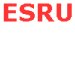MethodologyParametric Model   Power Profiles   Error Estimation

This section gives an overview of the method employed in the generation of the power profiles for the sites as well as assumptions used and the possible errors. The turbine parametric model used has also been included.

Parametric Model

The 1 MW design described in the Commercial Prospects of Tidal Stream document prepared by Binnie Black and Veatch in association with IT Power was adopted for this study. The concept is a twin rotor (2 x 500 kW) axial flow turbine mounted on a monopile founded in the seabed as shown below.

 Key characteristics of model Characteristic Units Value Rated power kW 1000 (2 x 500) Rated mechanical power kW 1120 Rated speed m/s 2.4 Cut-in speed m/s 0.7 Rotor rated speed rpm 14.4 Rotor Diameter m 15.85 Power Coefficient - 0.4 Gearbox transmission efficiency - 0.95 Generator efficiency - 0.94 Output voltage V 690Key features:
1. Variable-pitch mechanism allowing rotor blades to be adjusted through at least 1800so they can operate during both flood and ebb flows.
2. The twin rotors are contra-rotating to minimise resultant forces on the support structure.

Power Profiles

The sequence of steps involved in the generation of the power profiles is as below:
1. The speed variation with time was assumed to be sinusoidal completing a cycle every 12 hr 25 min. The maximum (reference) speed was taken as the mean of the mean neap and mean spring tides for both flood and ebb flow. The speeds were generated at one minute intervals. The chart tidal speeds were then compared to the sinusoidal speeds to estimate the level of accuracy.
2. Armed with the sinusoidal speed variation and a choice of turbine parameters the available power for each speed was calculated. The actual power was then found by multiplying the available power by the power coefficient taking into account the rated speed of the turbine. This was then converted to electrical power output by multiplying by the gearbox transmission efficiency as well as the generator efficiency.
3. The next step was the generation of power profiles (power output against time) for the various locations for a 12 hr 25mins cycle.
A spreadsheet was generated for each location which calculates the sinusoidal speeds, the available power, mechanical power output as well as the electrical power output at one minute intervals.

Error Estimation

The two main assumptions underlying the analysis are:
1. Sinusoidal variation of tidal speeds
2. 12 hr 25 min cycle repeating itself over time
Actual tidal speeds do not follow a sinusoidal variation and also do not always undergo a 12 hr 25 min cycle. The level of deviation from the actual tide behaviour is therefore crucial to the accuracy of the results. The purpose of this section is therefore to investigate the effects of these simplifying assumptions. The hourly tidal speeds from charts were superimposed with the sinusoidal speed variation and the deviation calculated by comparing the areas under the two graphs using the trapezium rule. It is worth noting that tidal data at one hour interval is not very indicative of true speed variation. A shorter time interval would have resulted in a better error estimation as the shape of the curve between two hourly points is approximate.